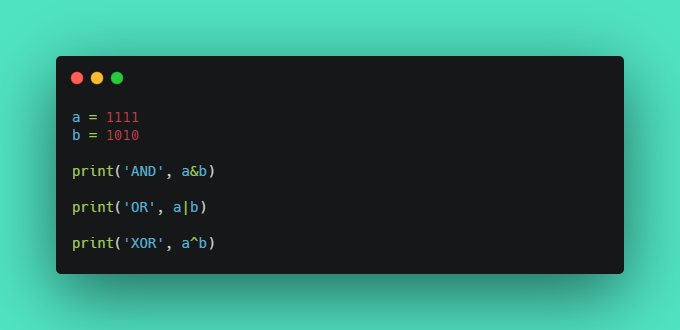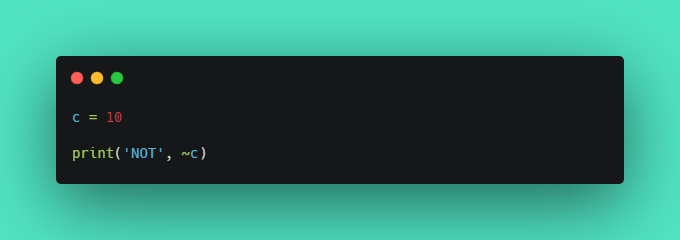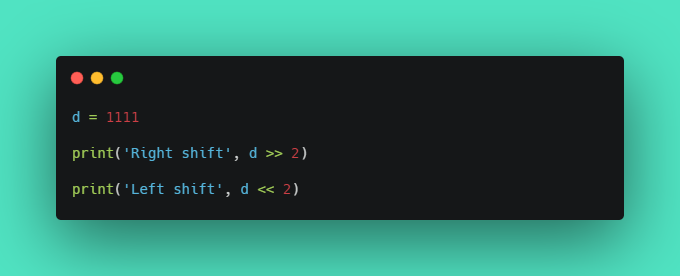Published on

# Bitwise Operators in python

Authors
•Name
Aswin Barath
@AswinBarath2

When it comes to binary numbers, bitwise operators are the choice.

Bitwise operators are used to performing operations on binary numbers.

## AND, OR, XOR operators

• AND `&` operator sets each bit to 1 if both bits are 1.
• OR `|` operator sets each bit to 1 if one of two bits is 1.
• XOR `^` operator sets each bit to 1 if only one of two bits is 1.Output:
``````AND 82
OR 2039
XOR 1957
``````

Ha Ha, surprised about the outputs?! The outputs are a result of the binary numbers a and b which gets converted into an integer, each time bitwise operation is performed.

## NOT operator

• NOT `~` operator inverts all the bits.
• In python, the number gets converted into an inverted signed number.Output:
``````NOT -11
``````

## Shift operators

• left shift `<<` operator shifts left by pushing zeros in from the right and let the leftmost bits fall off.
• right shift `>>` operator shifts right by pushing copies of the leftmost bit in from the left, and let the rightmost bits fall off.Output:
``````Right shift 277
Left shift 4444
``````